Courses

# Direction Sense Test

## 20 Questions MCQ Test Science Olympiad Class 4 | Direction Sense Test

Description
This mock test of Direction Sense Test for Class 4 helps you for every Class 4 entrance exam. This contains 20 Multiple Choice Questions for Class 4 Direction Sense Test (mcq) to study with solutions a complete question bank. The solved questions answers in this Direction Sense Test quiz give you a good mix of easy questions and tough questions. Class 4 students definitely take this Direction Sense Test exercise for a better result in the exam. You can find other Direction Sense Test extra questions, long questions & short questions for Class 4 on EduRev as well by searching above.
QUESTION: 1

Solution:
QUESTION: 2

Solution:
QUESTION: 3

### A rat runs 20 towards East and turns to right, runs 10 ft and turns to right, runs 9 ft and again turns to left, runs 5 ft and then turns to left, runs 12 ft and finally turns to left and runs 6 ft. Now, in which direction is the rat facing?

Solution:
QUESTION: 4

Starting from a point P, Sachin walked 20 metres towards South. He turned left and walked 30 metres. He then turned left and walked 20 metres . He again turned left and walked 40 metres and reached a point Q. How far and in which direction is the point Q from the point P?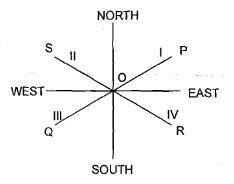Solution:

The distance from starting point will be PC−AB=40−30=10m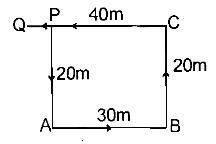QUESTION: 5

Rohan walks a distance of 3 Km towards North, then turns to his left and walks for 2 Km. He again turns left and walks for 3 Km. At this point he turns to his left and walks for 3 km. How many kilometers is he from the starting point?

Solution:
QUESTION: 6

A girl leaves from her home. She first walks 30 metres in North-west direction and then 30 metres in Southwest direction. Next, she walks 30 metres in south-east direction. Finally, she turns towards her house. In which direction is she moving?

Solution:
QUESTION: 7

Radha moves towards South-east, a distance of 7 Km, then she moves towards West and travels a distance of 14 m. From here, she moves towards North-west a distance of 7 m and finally she moves a distance of 4 m towards East and stood at that point. How far is the starting point from where she now stands?

Solution:

Radha’s movements are shown as below: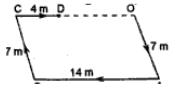Radha’s distance from starting point O = OD = (OC– CD) =(AB–CD) = (14– 4) m = 10 m

QUESTION: 8

A man is facing north-west. He turns 90° in the clockwise direction and then 135° in the anti clockwise direction. Which direction is he facing now?

Solution:
QUESTION: 9

You go North, turn right, then right again and then finally turn left. In which direction are you now?​

Solution:
QUESTION: 10

Namita walks 14 metres towards west, then turns to her right and walks 14 metres and then turns to her left and walks 10 metres. Again turning to her left she walks 14 metres. What is the shortest distance between her starting point and the present position?

Solution:

Namita’s movements of Namita are as shown in figure ( A to B, B to C, C to D and D to E). Clearly, Namita’s distance from his initial position = AE=(AB + BE)
=(AB + CD )
=(14 + 10)m = 24 m.

QUESTION: 11

A man is facing south. He turns 135° in the anti clockwise direction and then 180° in the clockwise direction. Which directions is he facing now?

Solution:
QUESTION: 12

Manick walked 40 metres towards North, took a left turn and walked 20 metres. He again took a left turn and walked 40 metres. How far and in which direction is he from the starting point?

Solution:
QUESTION: 13

Going 50 m to the south of her house, Radhika turns left and goes another 20 m. Then, turning to the North, she goes 30 m and then starts walking to her house. In which direction is she walking now?

Solution: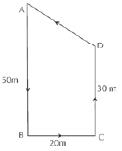Radhika’s movements are as shown in Fig. (A to B, B to C, C to D and D to A). Clearly, she is finally moving in the direction DA i.e. North

QUESTION: 14

Laxman went 15 km to the west from my house, then turned left and walked 20 Km. He then returned East and walked 25 Km and finally, turning left covered 20 Km. How far was he from his house?

Solution:
QUESTION: 15

Amit walked 30 metres towards East, took a right turn and walked 40 metres. Then he took a left turn and walked 30 metres. In which direction is he now from the starting point?

Solution:
QUESTION: 16

K is 40 m South-West of L. If M is 40 m South-East of L, then in which is M from K?

Solution: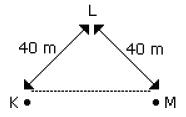Hence, M is in the East of K

QUESTION: 17

A man walks 2 Km towards North. Then he turns to East and walks 10 Km. After this he turns to North and walks 3 Km. Again he turns towards East and walks 2 Km. How far is he from the starting point?

Solution:
QUESTION: 18

The length and breadth of a room are 8 m and 6 m respectively. A cat runs along all the four walls and finally along a diagonal order to catch a rat. What is the total distance covered by the cat?

Solution:
QUESTION: 19

Sujata started to walk towards the rising Sun. After covering some distance she turned to right then again to the right and after covering some distance she again turns to the right. Which  direction is she facing?

Solution: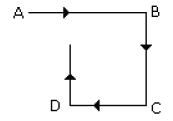Hence, finally Sujata will face towards North

QUESTION: 20

Some boys are sitting in three rows all facing North such that A is in the middle row. P is just to the right of A but in the same row. Q is just behind of P while R is in the North of A. In which direction of R is Q?

Solution: